﻿• 价格透明
• 信息保密
• 进度掌控
• 售后无忧# 【python技能树】python模块管理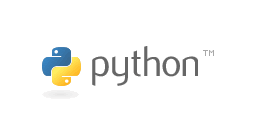# 🚩python模块导入安装教程

pip 是一个安装和管理 Python 包的工具。

pip的安装需要setuptools 或者 distribute，如果使用的是Python3.x那么就只能使用distribute，因为Python3.x不支持setuptools。

distribute下载地址： http://python-distribute.org/distribute_setup.py

1、解压pip安装包，在cmd中pip安装包路径下使用 python setup.py install

2、安装好后直接使用pip install xlrd 下载安装包即可

pip安装与换源下载见博文：手把手教你进行pip换源

# 🚩模块

• 1. 内置模块
• 2. 第三方模块
• 3. ⾃定义模块

## ⭐️为何要用模块

• 1. 使⽤内置或者第三⽅的模块的好处是：拿来主义，极⼤提升开发效率
• 2. 使⽤⾃定义的模块的好处是：将程序的各部分组件共⽤的功能提取出来放到⼀个模块中，其他的组件通过导入的方式使用该模块，该模块即⾃定义模块，好处是可以减少代码冗余。

## ⭐️导入模块

### 💗import + 模块

• 会产⽣⼀个模块名称空间
• 会执行模块⽂件的内容，将产⽣的名字丢到模的名称空间⾥
• 在当前执⾏的⽂件中拿到⼀个名字（模块名），该名字指向模块的名称空间

``module.func()``

import语句用于导入整个模块。

``````>>> import math	        #导入模块
>>> math.fabs(-5)	    #调用模块中的函数
5.0
>>> math.e		        #使用模块中的常量
2.718281828459045
>>> fabs(-5)			#试图直接使用模块中的函数，出错
Traceback (most recent call last):
File "<stdin>", line 1, in <module>
NameError: name 'fabs' is not defined``````

### 💗import + 模块 + as + 新名字

import语句用于导入整个模块，可用as为导入的模块指定一个新名称。 导入模块后，使用“模块名称.对象名称”格式来引用模块中的对象。

``````>>> import math as m	#导入模块并指定新名称
>>> m.fabs(-5)		    #通过新名称调用模块函数
5.0
>>> m.e			        #通过新名称使用模块常量
2.718281828459045``````

### 💗from + 模块 + import + 函数名，函数名，函数名……

• 会产生⼀个模块名称空间
• 会执行模块文件内容，见文件中产生的名字丢入到模块的名称空间中
• 在当前执行文件中拿到的函数名，该名字指向模块的名称空间中的函数

``func()``

from语句用于导入模块中的指定对象，导入的对象可直接使用，不需要使用模块名称作为限定符，示例代码如下：

``````>>> from math import fabs		#从模块导入指定函数
>>> fabs(-5)
5.0
>>> from math import e		#从模块导入指定常量
>>> e
2.718281828459045
>>> from math import fabs as f1	#导入时指定新名称
>>> f1(-10)
10.0

``````

from … import *语句使用星号时，可导入模块顶层的所有全局变量和函数 示例代码如下：

``````>>> from math import *	#导入模块顶层的全局变量和函数
>>> fabs(-5)			#直接使用导入的函数
5.0
>>> e			        #直接使用导入的常量
2.718281828459045

``````

## ⭐️导入时执行模块

import和from语句在执行导入操作时，会执行导入模块中的全部语句。

🍊import和from语句是隐性的赋值语句，两者的区别如下：

Python执行import语句时：

• 会创建一个模块对象和一个与模块文件同名的变量，并建立变量和模块对象的引用。
• 模块中的变量和函数等均作为模块对象的属性使用。
• 再次导入时，不会改变模块对象属性的当前值。

Python执行from语句时：

• 会同时在当前模块和被导入模块中创建同名变量，这两个变量引用同一个对象。
• 再次导入时，会将被导入模块的变量的初始值赋值给前模块的变量。

``````x=100					            #赋值，创建变量x
print('这是模块test.py中的输出！')	#输出字符串
def show():		                    #定义函数，执行时创建函数对象
print('这是模块test.py中的show()函数中的输出！')
``````

``````D:\>python
……
>>> import test		        #导入模块，下面的输出说明模块在导入时被执行

>>> test.x		            #使用模块变量
100
>>> test.x=200		        #为模块变量赋值
>>> import test		        #重新导入模块
>>> test.x		            #使用模块变量，输出结果显示重新导入未影响变量的值
200
>>> test.show()		        #调用模块函数

>>> abc=test			    #将模块变量赋值给另一个变量
>>> abc.x			        #使用模块变量
200
>>> abc.show()		        #调用模块函数

``````

🍊执行import导入后模块与变量的关系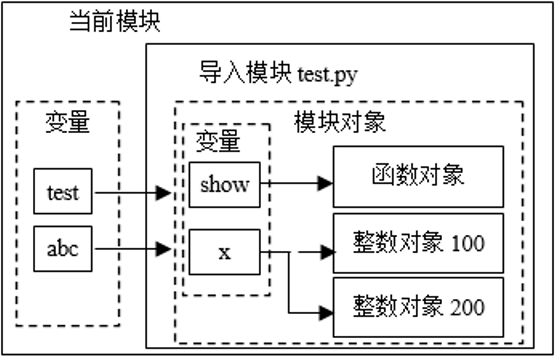``````>>> from test import x,show			#导入模块的变量x、show

>>> x						        #输出模块的变量的初始值
100
>>> show()					        #调用模块函数

>>> x=200					        #这里是为当前模块的变量赋值
>>> from test import x,show			#重新导入
>>> x						        #x的值为模块的变量的初始值
100
``````
• 在执行from语句时，test模块的所有语句均被执行。
• from语句将test模块的变量x和show赋值给当前模块的变量x和show。
• 语句“x=200”为当前模块的变量x赋值，不会影响test模块的变量x。
• 在重新导入时，当前模块变量x被重新赋值为test模块的变量x的值。

🍊执行from导入后模块与变量的关系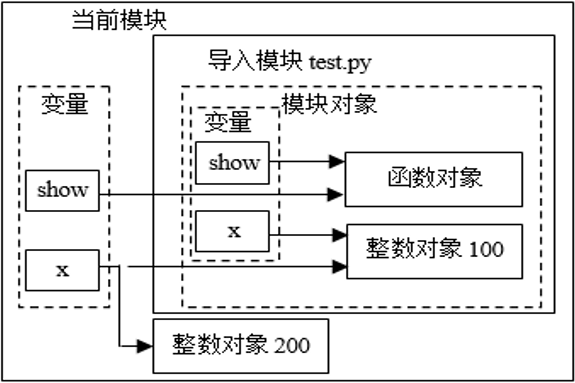## ⭐️用import还是from

### 💗使用模块内的可修改对象

🍊在下面的模块test中，变量x引用了整数对象100（100是不可修改对象），y引用了一个可修改的列表对象。

``````# test.py
x=100	    #赋值，创建整数对象100和变量x，变量x引用整数对象100
y=[10,20]	#赋值，创建列表对象[10,20]和变量y，变量y引用列表对象``````

🍊下面的代码使用from导入模块test

``````>>> x=10		        #创建当前模块的变量x
>>> y=[1,2]		        #创建当前模块的变量y
>>> from test import *	#引用模块中的x和y
>>> x,y			        #输出结果显示确实引用了模块中的对象
(100, [10, 20])
>>> x=200		        #赋值，使当前模块的变量x引用整数对象200
>>> y=['abc']		#修改第一个列表元素，此时会修改模块中的列表对象
>>> import test		    #再次导入模块
>>> test3.x,test.y	    #输出结果显示模块中的列表对象已被修改
(100, [['abc'], 20])

``````

### 💗使用from导入两个模块中的同名变量

🍊在下面的两个模块test1.pytest2.py中包含了同名的变量。

``````# test1.py
def show():
print('out in test1.py')

# test2.py
def show():
print('out in test2.py')``````

🍊当两个模块存在同名变量时，使用from语句导入模块会导致变量名冲突，示例代码如下：

``````>>> from test1 import show
>>> from test2 import show
>>> show()
out in test2.py
>>> from test2 import show
>>> from test1 import show
>>> show()
out in test1.py
``````

🍊当两个模块存在同名变量时，应使用import语句导入模块，示例代码如下。

``````>>> import test1
>>> import test2
>>> test1.show()
out in test1.py
>>> test2.show()
out in test2.py
``````

## ⭐️重新载入模块

``````>>> import test			#导入模块，模块代码被执行

>>> test.x
100
>>> test.x=200
>>> import test			#再次导入
>>> test.x				#再次导入没有改变当前值
200

<module 'test' from 'D:\\Python35\\test.py'>
>>> test.x		                #因为模块代码再次执行，x恢复为初始值
100
``````

## ⭐️模块搜索路径

🍊（1）搜索模块文件：在导入模块时， Python按特定的路径搜索模块文件。

🍊（2）必要时编译模块

• 找到模块文件后，Python会检查文件的时间戳。
• 如果字节码文件比源代码文件旧（即源代码文件作了修改），Python就会执行编译操作，生成最新的字节码文件。
• 如果字节码文件是最新的，则跳过编译环节。如果在搜索路径中只发现了字节码，而没有发现源代码文件，则直接加载字节码文件。
• 如果只有源代码文件，Python会执行编译操作，生成字节码文件。

🍊（3）执行模块：执行模块的字节码文件。嵌套导入模块查看模块对象属性__name__属性和命令行参数

``````>>> import sys
>>> sys.path
['', 'D:\\Python35\\python35.zip', 'D:\\Python35\\DLLs', 'D:\\Python35\\lib ', 'D:\\Python35', 'D:\\Python35\\lib\\site-packages']
``````

第一个空字符串表示Python当前工作目录。Python按照先后顺序依次搜索path列表中的路径。如果在path列表的所有路径中均未找到模块，则导入操作失败。

🍊（1）Python的当前工作目录（可用os模块中的getcwd()函数查看当前目录名称）。

🍊（2）操作系统的环境变量PYTHONPATH中包含的目录（如果存在）。

🍊（3）Python标准库目录。

🍊（4）任何pth文件包含的目录（如果有存在）。

Python按照上面的顺序搜索各个目录。

pth文件通常放在Python安装目录中，文件名可以任意，例如searchpath.pth

``````C:\myapp\hello
D:\pytemp\src
``````

sys.path列表在程序启动时，自动进行初始化。可在代码中对sys.path列表执行添加或删除操作

``````>>> from sys import path		#导入path变量
>>> path					    #显示当前搜索路径列表
……
>>> del path					#删除第二个搜索路径
>>> path
……
>>> path.append(r'D:\temp')		#添加一条搜索路径
>>> path
……
``````

## ⭐️PYTHONPATH 变量

``set PYTHONPATH=c:\python27\lib;``

``set PYTHONPATH=/usr/local/lib/python``

## ⭐️嵌套导入模块

Python允许任意层次的嵌套导入。

``````#test1.py
x=100
def show():
print('这是模块test.py中的show()函数中的输出！')
print('载入模块test.py！')
import test2

#test2.py
x2=200
print('载入模块test2.py！')
``````

``````>>> import test1				#导入模块test

>>> test1.x				        #使用test模块的变量
100
>>> test1.show()				#调用test模块的函数

>>> test1.test2.x2				#使用嵌套导入的test2模块中的变量
200

``````

## ⭐️查看模块对象属性

Python会为模块对象添加一些内置属性。

dir() 函数一个排好序的字符串列表，内容是一个模块里定义过的名字。

``````# 导入内置math模块
import math

content = dir(math)

print content;``````

``````['__doc__', '__file__', '__name__', 'acos', 'asin', 'atan',
'atan2', 'ceil', 'cos', 'cosh', 'degrees', 'e', 'exp',
'fabs', 'floor', 'fmod', 'frexp', 'hypot', 'ldexp', 'log',
'log10', 'modf', 'pi', 'pow', 'radians', 'sin', 'sinh',
'sqrt', 'tan', 'tanh']``````

## ⭐️__name__属性和命令行参数

``````#test.py
if __name__=='__main__':
#模块独立运行时，执行下面的代码
def show():
print('test.py独立运行')
show()
import sys
print(sys.argv)				        #输出命令行参数
else:
#作为导入模块时，执行下面的代码
def show():
print('test.py作为导入模块使用')
print('test.py执行完毕！')              	#该语句总会执行
``````

## ⭐️隐藏模块变量

from…import *”语句根据__all__列表导入变量名。只要是__all__列表中的变量，不管是否以下划线开头，均会被导入。

``````#test.py
x=100
_y=[1,2]
return a+b
def show():
print('out from test.py')
``````

# 🚩模块包

## ⭐️包的基本结构

__init__.py文件可以是一个空文件，或者在其中定义__all__列表、其他变量或类等。

🍊（1）打开Windows的资源管理器，在D盘根目录中新建文件夹pytemp。

🍊（2）在D:\pytemp中新建文件夹mypysrc。

🍊（3）在D:\pytemp\mypysrc中新建文件夹db。

🍊（4）在IDLE中创建一个空的Python程序，将其分别保存到D:\pytemp、D:\pytemp\mypysrc和D:\pytemp\mypysrc\db文件夹，命名为__init__.py。

🍊（5）在IDLE中创建一个Python程序，将其保存D:\pytemp\mypysrc\db文件夹，命名为test.py程序代码如下：

``````#D:\pytemp\mypysrc\db\test.py
def show():
print(r'这是模块D:\pytemp\mypysrc\db\test.py中的show()函数中的输出！')
print(r'模块D:\pytemp\mypysrc\db\test.py执行完毕！')``````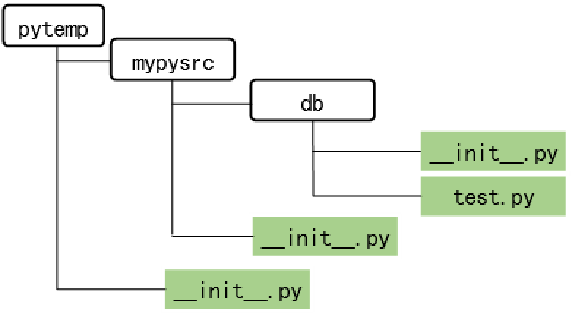## ⭐️导入包

``````D:\>cd pytemp		                        #进入包的根目录pytemp
D:\pytemp>python		                    #进入Python交互环境
……
>>>
>>> import mypysrc.db.test		            #导入包中的模块

>>> mypysrc.db.test.show()

>>> from mypysrc.db.test import show		#从包中的模块导入变量名
>>> show()

``````

## ⭐️相对导入

Python总是在搜索路径中查找包。

• “.”表示当前模块文件所在的目录——可称为当前路径，
• “..”表示当前模块文件所在路径的上一级目录。

### 💗使用当前路径导入

🍊在IDLE中创建一个Python程序，将其保存D:\pytem\mypysrc文件夹，命名为reltest.py。程序代码如下：

``````#D:\pytemp\mypysrc\reltest.py
import os
print('当前工作目录为：',os.getcwd())
from .db.test import show	  #导入当前目录下的db.test模块中的函数
show()
print('相对导入测试完毕')
``````

🍊在交互模式下导入reltest.py，示例代码如下：

``````>>> import mypysrc.reltest   #导入模块

### 💗使用上一级目录路径

🍊在IDLE中创建一个Python程序，将其保存D:\pytem\mypysrc文件夹，命名为test.py。程序代码如下：

``````#D:\pytemp\mypysrc\test.py
def show():
print(r'这是模块D:\pytemp\mypysrc\test.py中的show()函数中的输出！')
print(r'模块D:\pytemp\mypysrc\test.py执行完毕！')

``````

🍊在IDLE中创建一个Python程序，将其保存D:\pytem\mypysrc\db文件夹，命名为reltest_up.py。程序代码如下：

``````#D:\pytemp\mypysrc\db\reltest_up.py
from ..test import show	    #导入上一级目录下的test模块中的函数 show()
print(r'模块D:\pytemp\mypysrc\db\reltest_up.py执行完毕！')
print('相对导入测试完毕')
``````

🍊在交互模式下导入reltest_up.py，示例代码如下：

``````>>> import mypysrc.db.reltest_up

## ⭐️在__init__.py中添加代码

``````# __init__.py
import mypysrc.db.test
#__all__=['data1','showA']
data1='包d:\pytem\mypysrc中的变量data1的值'
data2='包d:\pytem\mypysrc中的变量data2的值'
def showA():
print('d:\pytem\mypysrc\__init__.py中的函数showA()的输出')
def showB():
print('d:\pytem\mypysrc\__init__.py中的函数showB()的输出')
print('D:\pytem\mypysrc\__init__.py执行完毕’)``````

# 🚩python常见模块与包

• 图片处理（PIL或Pillow）
• 网页解析（goose）
• 工具包下载安装（easy_install、pip）
• 数据库（MySQLdb）
• Web开发（Django）
• 机器学习（numpy、pandas、scipy）
• 游戏开发（PyGame）
• 文件操作（os）

# 🚩综合实例

## 👍杨辉三角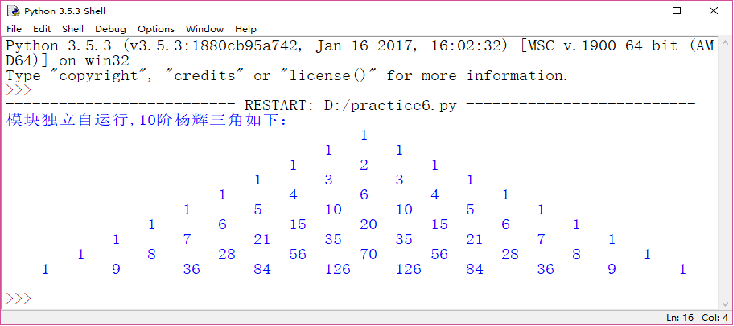🍊杨辉三角实现分析：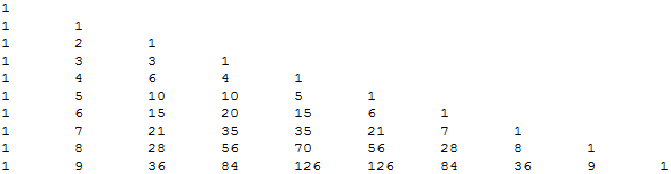• 第一列和主对角线上的数字都为1，
• 其他位置的数字为“上一行前一列”和“上一行同一列”两个位置的数字之和。
• 使用嵌套的列表表示杨辉三角，则非第一列和主对角线上元素的值可用下面的表达式表示。
• x[i][j]=x[i-1][j-1]+x[i-1][j]
``````输出：
第一行：L = 
len(L) = 1
range(1,1) = []    #此时， L[n-1] for n in range(1,len(L))] 这个for in 不执行
第二行：L =  + [L[n] + L[n-1] for n in [] ] + 
L =  + 
L = [1, 1]

len(L) = 2
range(1,2) = 
第三行：L =  + [L[n] + L[n-1] for n in ] + 
L =  + [ L + L[1-1] ] + 
L =  + [ L + L ] + 
L =  + [ 1 + 1] + 
L = [1, 2, 1]``````

``````def transfor(fun):   #定义一个转换函数，
def war(N):      #带参数的装饰器，把参数传闭包里
L = N.copy()
for j in range(len(L)):
temp = str(L[j])
L[j] = temp
l = ' '.join(L).center(90)
fun(l)
return war
@transfor
def f1(N):        #把print定义成f1()函数
print('模块独立运行，10阶杨辉三角如下：')
print(N)

N = 
for i in range(10):
f1(N)   #这里注意要用f()代替print()
N.append(0)
N = [N[k] + N[k-1] for k in range(i+2)]``````

🍊根据对称性打印（索引）：

``````n = 6
triangle = [,[1,1]]
for i in range(2,n):
row =  * (i+1)
for j in range(i//2):    #有图知：大概的临界值为一半，再仔细推敲
val = triangle[i-1][j] + triangle[i-1][j+1]
row[j+1] = val
row[i-j-1] =val      #此处规律：两个对称值的索引和等于 i
triangle.append(row)
print(triangle)

[, [1, 1], [1, 2, 1], [1, 3, 3, 1], [1, 4, 6, 4, 1], [1, 5, 10, 10, 5, 1]]``````

🍊单个列表覆盖、切片完成：

``````n = 6
row =  * n
for i in range(n):                #n行n次
old = 1                       #old刚开始为1
for j in range(i//2):
val = old + row[j+1]
old = row[j+1]            #此处现将row[j+1]的值赋给old，以防止被覆盖
row[j+1] = val
if i != 2*j:
row[i-j-1] = val
print(row[:i+1])


[1, 1]
[1, 2, 1]
[1, 3, 3, 1]
[1, 4, 6, 4, 1]
[1, 5, 10, 10, 5, 1]``````

## 👍包模块实例

``````PyPackage
│  PyCommonM.py
│  __init__.py
│
├─p1Package
│      P1M.py
│      P1MC.py
│      __init__.py
│
└─p2
P2.py
P2M.py``````

PyCommonM.py

``````def PyCommonMF():
print "PyCommonMF"``````

P1M.py

``````def P1MF():
print 'P1MF'``````

P1MC.py

``````class P1MC():
@staticmethod
def P1MCF():
print 'P1MCF'``````

P2M.py

``````def P2MF():
print 'P2MF'``````

P2.py

``````import P2M
from PyPackage import PyCommonM
from PyPackage.p1Package import P1M
from PyPackage.p1Package.P1MC import P1MC
def P2F():
print 'P2F'

if __name__ == '__main__':
P2F()
P2M.P2MF()
P1M.P1MF()
P1MC.P1MCF()
PyCommonM.PyCommonMF()``````

``````P2F
P2MF
P1MF
P1MCF
PyCommonMF``````

• 一个py文件为一module，例如module：PyCommonM.py，P2M.py，P1MC.py， P1M.py 。
• 包含__init__.py的文件夹为一个package,例如package： PyPackage，p1Package。
• 可以直接使用import来引用相同目录下的其他的module， 例如p2.py中的import P2M。
• 通过from import来引用其他的目录下且属于某个package的module，例如py.py中的from PyPackage import PyCommonM 和 from PyPackage.p1Package import P1M。
• 通过from import来引用某个module中的类，例如from PyPackage.p1Package.P1MC import P1MC

### 👍批量处理图片   PIL(Image、ImageDraw、ImageFont)、os、time（时间戳）

``````import os,time
from PIL import Image,ImageDraw,ImageFont

#源文件夹，目标文件夹，字体文件，屏幕分辨率（宽度，高度）
srcpath, destpath, fontfile = r".\src", r"..\dest", r"..\font\301-CAI978.ttf"
width, height = 640.0, 1136.0
def zoomSize(filename,imgtype):
imgtype = "jpeg" if imgtype=="jpg" else "png"
#获取文件创建时间        例：1483882912.37   Sun Jan 08 21:41:52 2017
t= time.ctime(os.path.getctime(filename))
print filename +" 创建时间:"+ t
im = Image.open(filename)   #默认mode="r"
#在图片上添加创建时间
draw = ImageDraw.Draw(im)
fontsize = min(im.size)/30
font = ImageFont.truetype(fontfile, fontsize)
draw.text((0,im.height - fontsize),t,font=font,fill=(255,0,0))
#按比例缩放，使之适应屏幕分辨率
rate = max( im.width/width, im.height/height )
if rate!=0:
im.thumbnail((im.size/rate , im.size/rate))    #注：此处有两个括号,thumbnail的处理对象是元组
return im

def batchZoomSize():
imgBatch = {}　　　　#字典  {文件名:对应的处理后Image对象}
#切换至源文件目录，对每张图片进行处理
os.chdir(srcpath)
for filename in os.listdir(os.getcwdu()):    #列举当前目录下的所有文件
postfix = os.path.splitext(filename)  #获取元祖  (文件名,文件后缀)
if postfix == ".jpg" or postfix == ".png":
imgBatch[filename]=zoomSize(filename, postfix)
#切换至目标文件目录，保存图片至目标文件夹,关闭图片文件
os.chdir(destpath)
for filename in imgBatch.keys():
imgBatch[filename].save(filename)
imgBatch[filename].close()``````

### 👍生成序列号　　random（随机数）、string（字符串）

``````import string,random
field = string.letters + string.digits    #'abcdefghijklmnopqrstuvwxyzABCDEFGHIJKLMNOPQRSTUVWXYZ0123456789'

def getRandom(size):
return "".join(random.sample(field,size))

def generate(n,group,size):
for i in range(n):
"-".join([getRandom(size) for i in range(group)])　　#注意：()里面还有[]，join的处理对象时列表

if __name__=="__main__":
print generate(2,4,4)            #['YcwC-fzE4-93pl-ROnY', '76AI-h1Aa-BNK4-PuFR']``````

### 👍统计单词词频　　os、re（正则表达式）、collections.Counter（统计计数）

``````import os,re
from collections import Counter'''

例：Counter({word1:num1,word2:num2})
'''
def getCount(filename):
pattern = r'''[A-Za-z]+|\\$?\d+%?\$'''
with open(filename) as f:
return Counter(r)
'''

'''
def saveFile(filename,counter):
fp = open(filename,"w")for t in counter:
fp.write(bytes(t) + "," + bytes(t)+"\n")    # 单词,词频
fp.close()

count = getCount(r".\doc\1.txt")
saveFile("count.csv",count.most_common())``````### 低价透明### 金牌服务### 信息保密